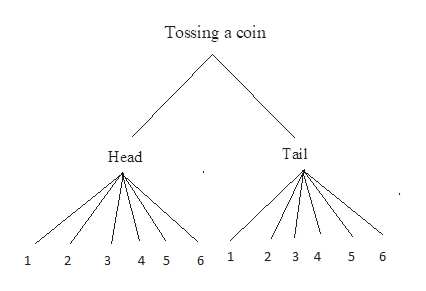Consider the following. (a) Draw a tree diagram to display all the possible outcomes that can occur when you flip a coin and then toss a die. (b) How many outcomes contain a head and a number greater than 4?(c) Probability extension: Assuming the outcomes displayed in the tree diagram are all equally likely, what is the probability that you will get a head and a number greater than 4 when you flip a coin and toss a die? (Round your answer to three decimal places.)

Question

Consider the following.
(a) Draw a tree diagram to display all the possible outcomes that can occur when you flip a coin and then toss a die.

(b) How many outcomes contain a head and a number greater than 4?

(c) Probability extension: Assuming the outcomes displayed in the tree diagram are all equally likely, what is the probability that you will get a head and a number greater than 4 when you flip a coin and toss a die? (Round your answer to three decimal places.)

Step 1

a).

The required tree diagram is gi...help_outlineImage TranscriptioncloseTossing a coin Tail Head 2 3 4 5 6 12 3 4 5 6 1 fullscreen

Want to see the full answer?

See Solution

Want to see this answer and more?

Our solutions are written by experts, many with advanced degrees, and available 24/7

See Solution
Tagged in

Basic Probability